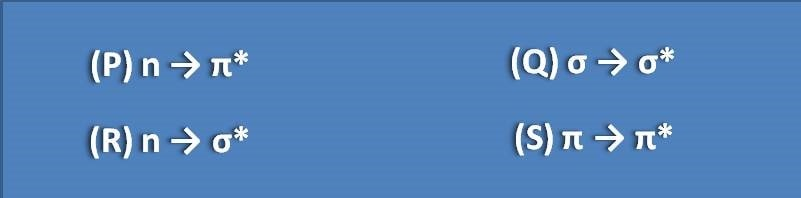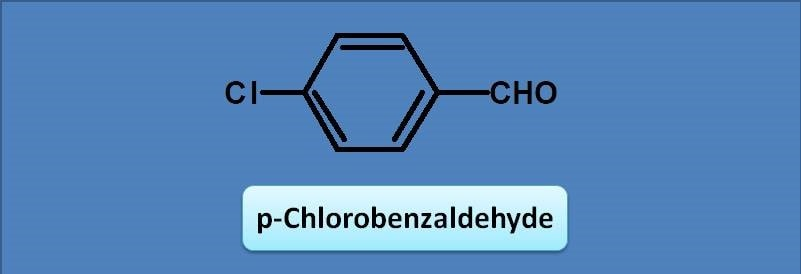# MCQ on UV-Visible spectroscopy: Page-2

1. The base value for teh following compound is(A) 246 nm

(B) 250 nm

(C) 230 nm

(D) 217 nm

The given structure is 1,3-pentadiene and since it has pi bonds it can undergo pi to pi transition. Therefore the diene acts as chromophore in this molecule with a base value of 217 nm.

2. The approximate lambda max for the above compound will be

(A) 214 nm

(B) 217 nm

(C) 222 nm

(D) 227 nm

First let’s give numbering to the chromophore. Here diene is the chromophore which has a base value as 217 nm. Now let’s see any groups attached to this chromophore. One methyl group is present at 4th position, so we have to give an increment of 5 nm. Combining these, the total value for lambda max is 217 + 5=222 nm

3. The possible transitions for water molecule in UV-visible region are

(A) σ →σ *

(B) n→π *, π→π *

(C) σ→σ *, n→π *

(D) n → σ*

Water has sigma and n electrons. So it can show sigma to sigma and n to sigma transitions. These two types of transitions fall under vacuum UV hence water doesn’t absorb radiation within the UV-Visible region.

4. Energies required for the following transitions in increasing order(A) P<Q<R<S

(B) Q<S<R<P

(C) S<R<Q<P

(D) P<S<R<Q

The energy required for a transition depends on the energy gap between the bonding molecular orbital and anti-bonding molecular orbital of the particular electron. For example, the energy gap is highest between sigma and sigma* requiring more energy for this transition. Similarly, the energy gap is least between n electrons and pi*.Note that electrons that form either sigma or pi bond only will have anti-bonding molecular orbital. n electrons are not involved in bonding hence don’t have excited state.

5. Base value for the following compound will be(A) 230

(B) 250

(D) 246

(D) 217

Since the chromophore is benzaldehyde, the base value will be 250 nm according to Woodward-Fieser rules.